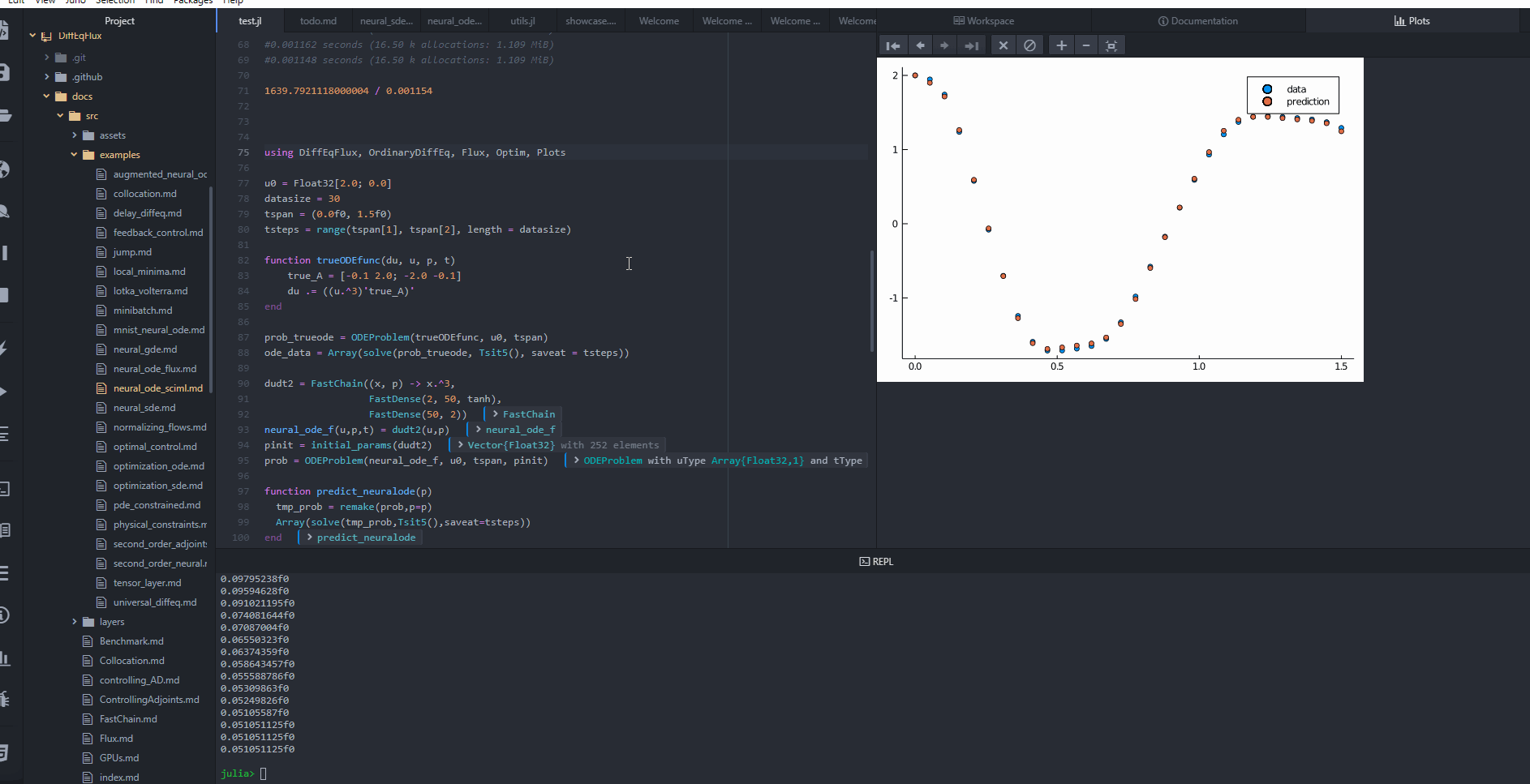# Neural Ordinary Differential Equations

A neural ODE is an ODE where a neural network defines its derivative function. Thus for example, with the multilayer perceptron neural network Lux.Chain(Lux.Dense(2, 50, tanh), Lux.Dense(50, 2)), we can define a differential equation which is u' = NN(u). This is done simply by the NeuralODE struct. Let's take a look at an example.

## Copy-Pasteable Code

Before getting to the explanation, here's some code to start with. We will follow a full explanation of the definition and training process:

using Lux, DiffEqFlux, DifferentialEquations, Optimization, OptimizationOptimJL, Random, Plots

rng = Random.default_rng()
u0 = Float32[2.0; 0.0]
datasize = 30
tspan = (0.0f0, 1.5f0)
tsteps = range(tspan, tspan, length = datasize)

function trueODEfunc(du, u, p, t)
true_A = [-0.1 2.0; -2.0 -0.1]
du .= ((u.^3)'true_A)'
end

prob_trueode = ODEProblem(trueODEfunc, u0, tspan)
ode_data = Array(solve(prob_trueode, Tsit5(), saveat = tsteps))

dudt2 = Lux.Chain(x -> x.^3,
Lux.Dense(2, 50, tanh),
Lux.Dense(50, 2))
p, st = Lux.setup(rng, dudt2)
prob_neuralode = NeuralODE(dudt2, tspan, Tsit5(), saveat = tsteps)

function predict_neuralode(p)
Array(prob_neuralode(u0, p, st))
end

function loss_neuralode(p)
pred = predict_neuralode(p)
loss = sum(abs2, ode_data .- pred)
return loss, pred
end

# Do not plot by default for the documentation
# Users should change doplot=true to see the plots callbacks
callback = function (p, l, pred; doplot = false)
println(l)
# plot current prediction against data
plt = scatter(tsteps, ode_data[1,:], label = "data")
scatter!(plt, tsteps, pred[1,:], label = "prediction")
if doplot
display(plot(plt))
end
return false
end

pinit = Lux.ComponentArray(p)
callback(pinit, loss_neuralode(pinit)...; doplot=true)

# use Optimization.jl to solve the problem

optf = Optimization.OptimizationFunction((x, p) -> loss_neuralode(x), adtype)
optprob = Optimization.OptimizationProblem(optf, pinit)

result_neuralode = Optimization.solve(optprob,
callback = callback,
maxiters = 300)

optprob2 = remake(optprob,u0 = result_neuralode.u)

result_neuralode2 = Optimization.solve(optprob2,
Optim.BFGS(initial_stepnorm=0.01),
callback=callback,
allow_f_increases = false)

callback(result_neuralode2.u, loss_neuralode(result_neuralode2.u)...; doplot=true)
false## Explanation

Let's get a time series array from a sprial ODE to train against.

using Lux, DiffEqFlux, DifferentialEquations, Optimization, OptimizationOptimJL, Random, Plots

rng = Random.default_rng()
u0 = Float32[2.0; 0.0]
datasize = 30
tspan = (0.0f0, 1.5f0)
tsteps = range(tspan, tspan, length = datasize)

function trueODEfunc(du, u, p, t)
true_A = [-0.1 2.0; -2.0 -0.1]
du .= ((u.^3)'true_A)'
end

prob_trueode = ODEProblem(trueODEfunc, u0, tspan)
ode_data = Array(solve(prob_trueode, Tsit5(), saveat = tsteps))
2×30 Matrix{Float32}:
2.0  1.9465    1.74178  1.23837  0.577127  …  1.40688   1.37023   1.29214
0.0  0.798832  1.46473  1.80877  1.86465      0.451377  0.728699  0.972102

Now let's define a neural network with a NeuralODE layer. First we define the layer. Here we're going to use Lux.Chain, which is a suitable neural network structure for NeuralODEs with separate handling of state variables:

dudt2 = Lux.Chain(x -> x.^3,
Lux.Dense(2, 50, tanh),
Lux.Dense(50, 2))
p, st = Lux.setup(rng, dudt2)
prob_neuralode = NeuralODE(dudt2, tspan, Tsit5(), saveat = tsteps)
NeuralODE()         # 252 parameters

Note that we can directly use Chains from Flux.jl as well, for example:

dudt2 = Chain(x -> x.^3,
Dense(2, 50, tanh),
Dense(50, 2))

In our model we used the x -> x.^3 assumption in the model. By incorporating structure into our equations, we can reduce the required size and training time for the neural network, but a good guess needs to be known!

From here we build a loss function around it. The NeuralODE has an optional second argument for new parameters which we will use to iteratively change the neural network in our training loop. We will use the L2 loss of the network's output against the time series data:

function predict_neuralode(p)
Array(prob_neuralode(u0, p, st))
end

function loss_neuralode(p)
pred = predict_neuralode(p)
loss = sum(abs2, ode_data .- pred)
return loss, pred
end
loss_neuralode (generic function with 1 method)

We define a callback function. In this example we set doplot = false because otherwise it would show every step and overflow the documentation, but for your use case set doplot=true to see a live animation of the training process!.

# Callback function to observe training
callback = function (p, l, pred; doplot = false)
println(l)
# plot current prediction against data
plt = scatter(tsteps, ode_data[1,:], label = "data")
scatter!(plt, tsteps, pred[1,:], label = "prediction")
if doplot
display(plot(plt))
end
return false
end

pinit = Lux.ComponentArray(p)
callback(pinit, loss_neuralode(pinit)...)
false

We then train the neural network to learn the ODE.

Here we showcase starting the optimization with ADAM to more quickly find a minimum, and then honing in on the minimum by using LBFGS. By using the two together, we are able to fit the neural ODE in 9 seconds! (Note, the timing commented out the plotting). You can easily incorporate the procedure below to set up custom optimization problems. For more information on the usage of Optimization.jl, please consult this documentation.

The x and p variables in the optimization function are different than x and p above. The optimization function runs over the space of parameters of the original problem, so x_optimization == p_original.

# Train using the ADAM optimizer

optf = Optimization.OptimizationFunction((x, p) -> loss_neuralode(x), adtype)
optprob = Optimization.OptimizationProblem(optf, pinit)

result_neuralode = Optimization.solve(optprob,
callback = callback,
maxiters = 300)
u: ComponentVector{Float32}(layer_1 = Float32[], layer_2 = (weight = Float32[-0.13110653 -1.7518417; 0.42772067 -0.39959562; … ; -0.09926208 0.62332004; 0.53811353 0.8318834], bias = Float32[0.40514722; 0.09246531; … ; -0.79016745; 0.54765916;;]), layer_3 = (weight = Float32[0.18856353 0.6770037 … -0.4877874 -0.5770116; -0.4236453 0.16645837 … -0.10709219 -0.36172003], bias = Float32[-0.5798364; 0.06284115;;]))

We then complete the training using a different optimizer starting from where ADAM stopped. We do allow_f_increases=false to make the optimization automatically halt when near the minimum.

# Retrain using the LBFGS optimizer
optprob2 = remake(optprob,u0 = result_neuralode.u)

result_neuralode2 = Optimization.solve(optprob2,
Optim.BFGS(initial_stepnorm=0.01),
callback = callback,
allow_f_increases = false)
u: ComponentVector{Float32}(layer_1 = Float32[], layer_2 = (weight = Float32[-0.18714459 -1.6713033; 0.39644974 -0.26139396; … ; -0.09197057 0.5915809; 0.1609493 1.0725534], bias = Float32[0.5926635; 0.11802127; … ; -0.8342508; 0.43537012;;]), layer_3 = (weight = Float32[-0.012222614 0.6856742 … -0.6441489 -0.597344; -0.30141473 0.19221112 … -0.29129496 -0.4125885], bias = Float32[-0.34279087; 0.60657835;;]))

And then we use the callback with doplot=true to see the final plot:

callback(result_neuralode2.u, loss_neuralode(result_neuralode2.u)...; doplot=true)
false